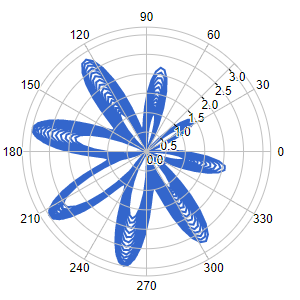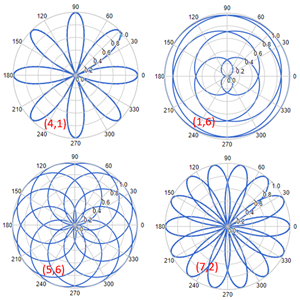### Free Ginckers

#### Polar Chart: cos(a*t)^3 + sin(b*t)^2

Template: Graphics/polar-chart

Discription: A polar chart is a circular plot on which data points are displayed using the angle (t), and the distance (r) from the center point.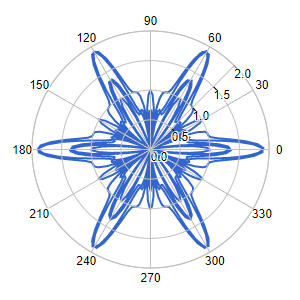#### Polar Chart: Log(Cosine), Log(Sine)

Template: Graphics/polar-chart

Discription: A polar chart is a circular plot on which data points are displayed using the angle (t), and the distance (r) from the center point.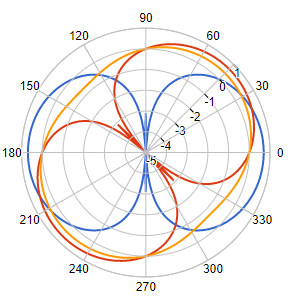#### Polar Chart: Sine 10

Template: Graphics/polar-chart

Discription: A polar chart is a circular plot on which data points are displayed using the angle (t), and the distance (r) from the center point.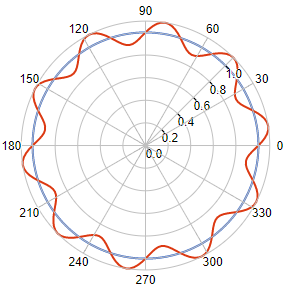#### Polar Chart: Sine40-39

Template: Graphics/polar-chart

Discription: A polar chart is a circular plot on which data points are displayed using the angle (t), and the distance (r) from the center point.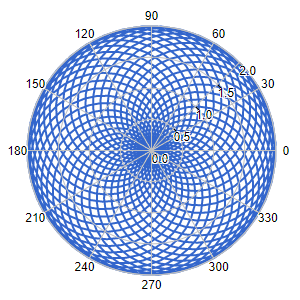#### Polar Chart: SIne50-49

Template: Graphics/polar-chart

Discription: A polar chart is a circular plot on which data points are displayed using the angle (t), and the distance (r) from the center point.